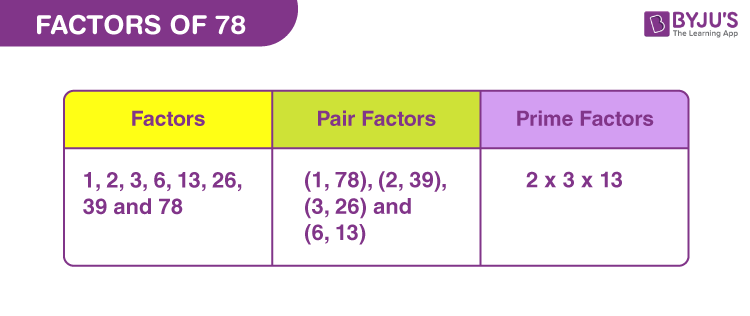# Factors of 78

In Mathematics, factors of 78 are the natural numbers that can divide the original number, uniformly. Alternatively, when the product of two natural numbers is equal to 78, then the two numbers are the required factors.

Factors of 78 break the original number into equal parts. If we divide 78 by any of its factors, then the resultant quotient is a whole number and remainder is zero. Since 78 is an even number, thus it is also divisible by 2. Hence, 78 is a composite number and has more than two factors that include 1, 2 and 79. To find more factors, let us read this article.## How to Find the Factors of 78?

Factors of 78 divide the original number, exactly. We know all the numbers are the factors of itself and also 1 is the factor of all the numbers. Therefore, the two definite factors of 78 are 1 and 78. Now, let us find the other factors.

78 ÷ 1 = 78

78 ÷ 2 = 39

78 ÷ 3 = 26

78 ÷ 6 = 13

78 ÷ 13 = 6

78 ÷ 26 = 3

78 ÷ 39 = 2

78 ÷ 78 = 1

Therefore, the factors of 78 are 1, 2, 3, 6, 13, 26, 39, and 78.

## Pair Factors of 78

When two integers are multiplied and the product is 78, then the pair of integers are called pair factors of 78.

1 × 78 = 78

2 × 39 = 78

3 × 26 = 78

6 × 13 = 78

Therefore, the pair factors are (1, 78), (2, 39), (3, 26), and (6, 13).

Similarly, we can write the negative pair factors that will result in the original number, after multiplication.

-1 × -78 = 78

-2 × -39 = 78

-3 × -26 = 78

-6 × -13 = 78

Therefore, the negative pair factors are (-1, -78), (-2, -39), (-3, -26), and (-6, -13).

## Prime Factorisation of 78

Prime factorisation of 78 represents the multiplication of prime numbers that will be equal to the original number. We need to check the prime numbers, 78 is divisible by, to find the prime factors of 78.

List of prime numbers (Between 1 and 78) :

 2, 3, 5, 7, 11, 13, 17, 19, 23, 29, 31, 37, 41, 43, 47, 53, 59, 61, 67, 71, 73

Step 1: 78 is an even number. Thus, it is divisible by 2.

78/2 = 39

Step 2: Since 39 is divisible by 3, thus;

39/3 = 13

Step 3: Now 13 is itself a prime number. Therefore,

13/13 = 1

Now 1 is not divisible by any prime number. Thus, the required prime factors are 2, 3 and 13.

 Prime factorisation of 78 = 2 x 3 x 13 Exponential Form = 21 x 31 x 131

## Solved Examples

Q.1: What is the value of 78 divided by 13?

Solution: 78 divided by 13 = 78 ÷ 13 = 6

Q.2: Find the sum of all the factors of 78.

Solution: The factors of 78 are 1, 2, 3, 6, 13, 26, 39, and 78 .

Sum = 1 + 2 + 3 + 6 + 13 + 26 + 39 + 78 = 168

Therefore, the sum of factors of 78 is 168.

Q.3: Find the greatest common factor of 70 and 78.

Answer: Let us first write the factors of both the numbers.

70 → 1, 2, 5, 7, 10, 14, 35, 70

78 → 1, 2, 3, 6, 13, 26, 39, 78

Therefore, greatest common factor (GCF) of 70 and 78 = 2

### Practice Questions

1. What is the average of factors of 78?

2. Arrange the factors of 78 in descending order.

3. Find if 6 is a factor of 78.

4. Calculate the GCF of 70, 72, 76 and 78.

5. Give any two pair factors of 78.

## Frequently Asked Questions on Factors of 78

### What are the factors of 78?

There are a total eight factors of 78, they are 1, 2, 3, 6, 13, 26, 39, and 78.

### What is the prime factors form of 78?

The prime factorisation of 78 is: 2 x 3 x 13.

### What is the greatest prime factor of 78?

The greatest prime factor of 78 is 13.

### What are the multiples of 78?

The first ten multiples of 78 are 78, 156, 234, 312, 390, 468, 546, 624, 702 and 780.

### Is 78 a prime or a composite number?

78 is a composite number, since it has a total of eight factors but to be a prime number, the total factors should be only two.